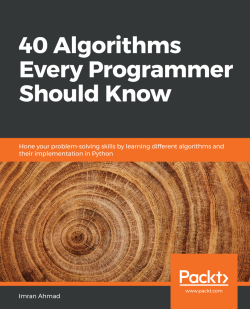# 40 Algorithms Every Programmer Should Know4.5 (20 reviews total)
• \$28.99 eBook
• \$41.99 Print + eBook
What do you get with a Packt Subscription?

• Instant access to this title and 7,500+ eBooks & Videos
• Constantly updated with 100+ new titles each month
• Breadth and depth in over 1,000+ technologies

Algorithms have always played an important role in both the science and practice of computing. Beyond traditional computing, the ability to use algorithms to solve real-world problems is an important skill that any developer or programmer must have. This book will help you not only to develop the skills to select and use an algorithm to solve real-world problems but also to understand how it works.

You’ll start with an introduction to algorithms and discover various algorithm design techniques, before exploring how to implement different types of algorithms, such as searching and sorting, with the help of practical examples. As you advance to a more complex set of algorithms, you'll learn about linear programming, page ranking, and graphs, and even work with machine learning algorithms, understanding the math and logic behind them. Further on, case studies such as weather prediction, tweet clustering, and movie recommendation engines will show you how to apply these algorithms optimally. Finally, you’ll become well versed in techniques that enable parallel processing, giving you the ability to use these algorithms for compute-intensive tasks.

By the end of this book, you'll have become adept at solving real-world computational problems by using a wide range of algorithms.

Publication date:
June 2020
Publisher
Packt
Pages
382
ISBN
9781789801217

# Overview of Algorithms

This book covers the information needed to understand, classify, select, and implement important algorithms. In addition to explaining their logic, this book also discusses data structures, development environments, and production environments that are suitable for different classes of algorithms. We focus on modern machine learning algorithms that are becoming more and more important. Along with the logic, practical examples of the use of algorithms to solve actual everyday problems are also presented.

This chapter provides an insight into the fundamentals of algorithms. It starts with a section on the basic concepts needed to understand the workings of different algorithms. This section summarizes how people started using algorithms to mathematically formulate a certain class of problems. It also mentions the limitations of different algorithms. The next section explains the various ways to specify the logic of an algorithm. As Python is used in this book to write the algorithms, how to set up the environment to run the examples is explained. Then, the various ways that an algorithm's performance can be quantified and compared against other algorithms are discussed. Finally, this chapter discusses various ways a particular implementation of an algorithm can be validated.

To sum up, this chapter covers the following main points:

• What is an algorithm?

• Specifying the logic of an algorithm

• Introducing Python packages

• Algorithm design techniques

• Performance analysis

• Validating an algorithm

# What is an algorithm?

In the simplest terms, an algorithm is a set of rules for carrying out some calculations to solve a problem. It is designed to yield results for any valid input according to precisely defined instructions. If you look up the word algorithm in an English language dictionary (such as American Heritage), it defines the concept as follows:

"An algorithm is a finite set of unambiguous instructions that, given some set of initial conditions, can be performed in a prescribed sequence to achieve a certain goal and that has a recognizable set of end conditions."

Designing an algorithm is an effort to create a mathematical recipe in the most efficient way that can effectively be used to solve a real-world problem. This recipe may be used as the basis for developing a more reusable and generic mathematical solution that can be applied to a wider set of similar problems.

# The phases of an algorithm

The different phases of developing, deploying, and finally using an algorithm are illustrated in the following diagram: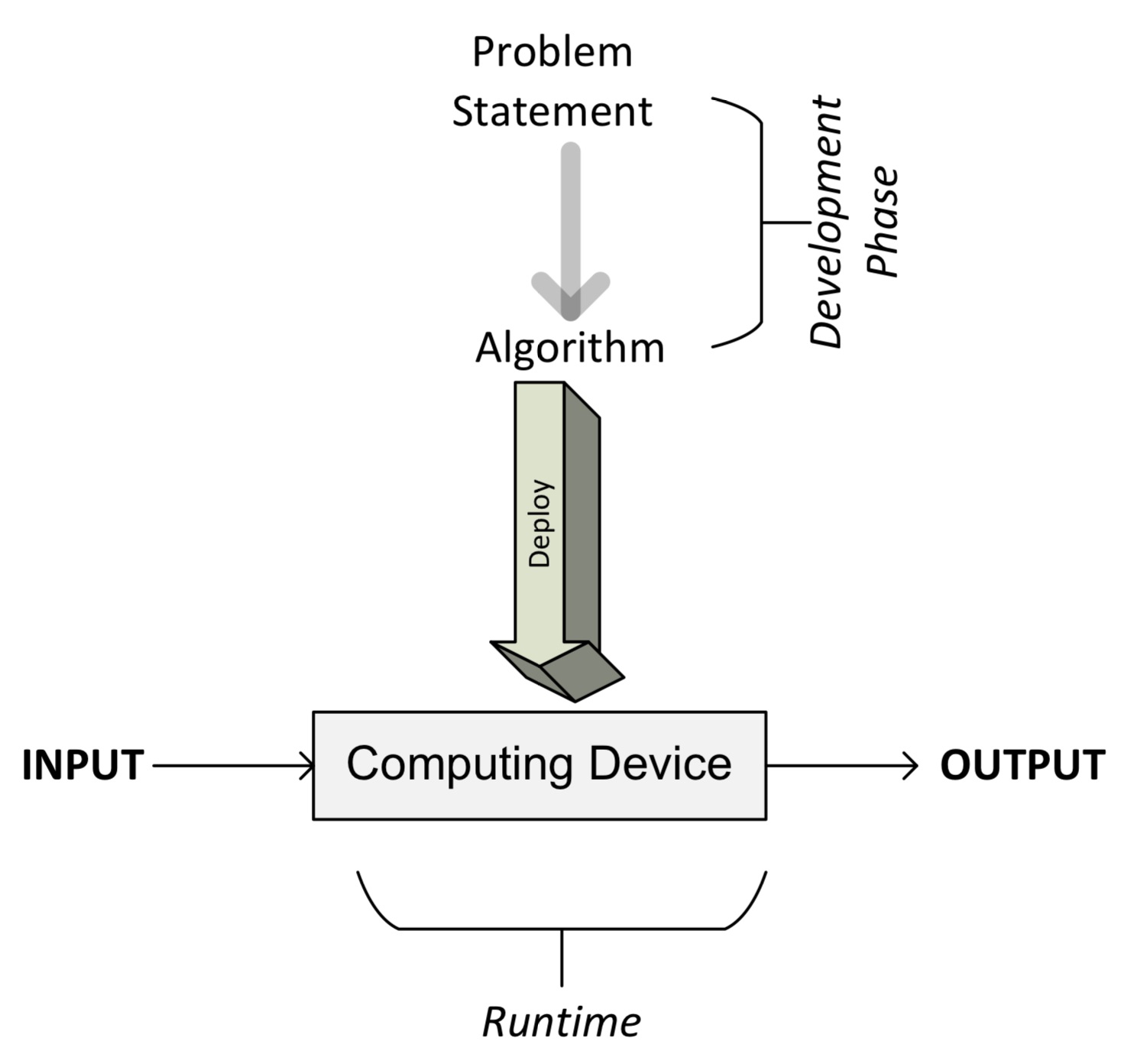As we can see, the process starts with understanding the requirements from the problem statement that detail what needs to be done. Once the problem is clearly stated, it leads us to the development phase.

The development phase consists of two phases:

• The design phase: In the design phase, the architecture, logic, and implementation details of the algorithm are envisioned and documented. While designing an algorithm, we keep both accuracy and performance in mind. While searching for the solution to a given problem, in many cases we will end up having more than one alternative algorithm. The design phase of an algorithm is an iterative process that involves comparing different candidate algorithms. Some algorithms may provide simple and fast solutions but may compromise on accuracy. Other algorithms may be very accurate but may take considerable time to run due to their complexity. Some of these complex algorithms may be more efficient than others. Before making a choice, all the inherent tradeoffs of the candidate algorithms should be carefully studied. Particularly for a complex problem, designing an efficient algorithm is really important. A correctly designed algorithm will result in an efficient solution that will be capable of providing both satisfactory performance and reasonable accuracy at the same time.

• The coding phase: In the coding phase, the designed algorithm is converted into a computer program. It is important that the actual program implements all the logic and architecture suggested in the design phase.

The designing and coding phases of an algorithm are iterative in nature. Coming up with a design that meets both functional and non-functional requirements may take lots of time and effort. Functional requirements are those requirements that dictate what the right output for a given set of input data is. Non-functional requirements of an algorithm are mostly about the performance for a given size of data. Validation and performance analysis of an algorithm are discussed later in this chapter. Validating an algorithm is about verifying that an algorithm meets its functional requirements. Performance analysis of an algorithm is about verifying that it meets its main non-functional requirement: performance.

Once designed and implemented in a programming language of your choice, the code of the algorithm is ready to be deployed. Deploying an algorithm involves the design of the actual production environment where the code will run. The production environment needs to be designed according to the data and processing needs of the algorithm. For example, for parallelizable algorithms, a cluster with an appropriate number of computer nodes will be needed for the efficient execution of the algorithm. For data-intensive algorithms, a data ingress pipeline and the strategy to cache and store data may need to be designed. Designing a production environment is discussed in more detail in Chapter 13Large Scale Algorithms, and Chapter 14Practical Considerations. Once the production environment is designed and implemented, the algorithm is deployed, which takes the input data, processes it, and generates the output as per the requirements.

# Specifying the logic of an algorithm

When designing an algorithm, it is important to find different ways to specify its details. The ability to capture both its logic and architecture is required. Generally, just like building a home, it is important to specify the structure of an algorithm before actually implementing it. For more complex distributed algorithms, pre-planning the way their logic will be distributed across the cluster at running time is important for the iterative efficient design process. Through pseudocode and execution plans, both these needs are fulfilled and are discussed in the next section.

# Understanding pseudocode

The simplest way to specify the logic for an algorithm is to write the higher-level description of an algorithm in a semi-structured way, called pseudocode. Before writing the logic in pseudocode, it is helpful to first describe its main flow by writing the main steps in plain English. Then, this English description is converted into pseudocode, which is a structured way of writing this English description that closely represents the logic and flow for the algorithm. Well-written algorithm pseudocode should describe the high-level steps of the algorithm in reasonable detail, even if the detailed code is not relevant to the main flow and structure of the algorithm. The following figure shows the flow of steps: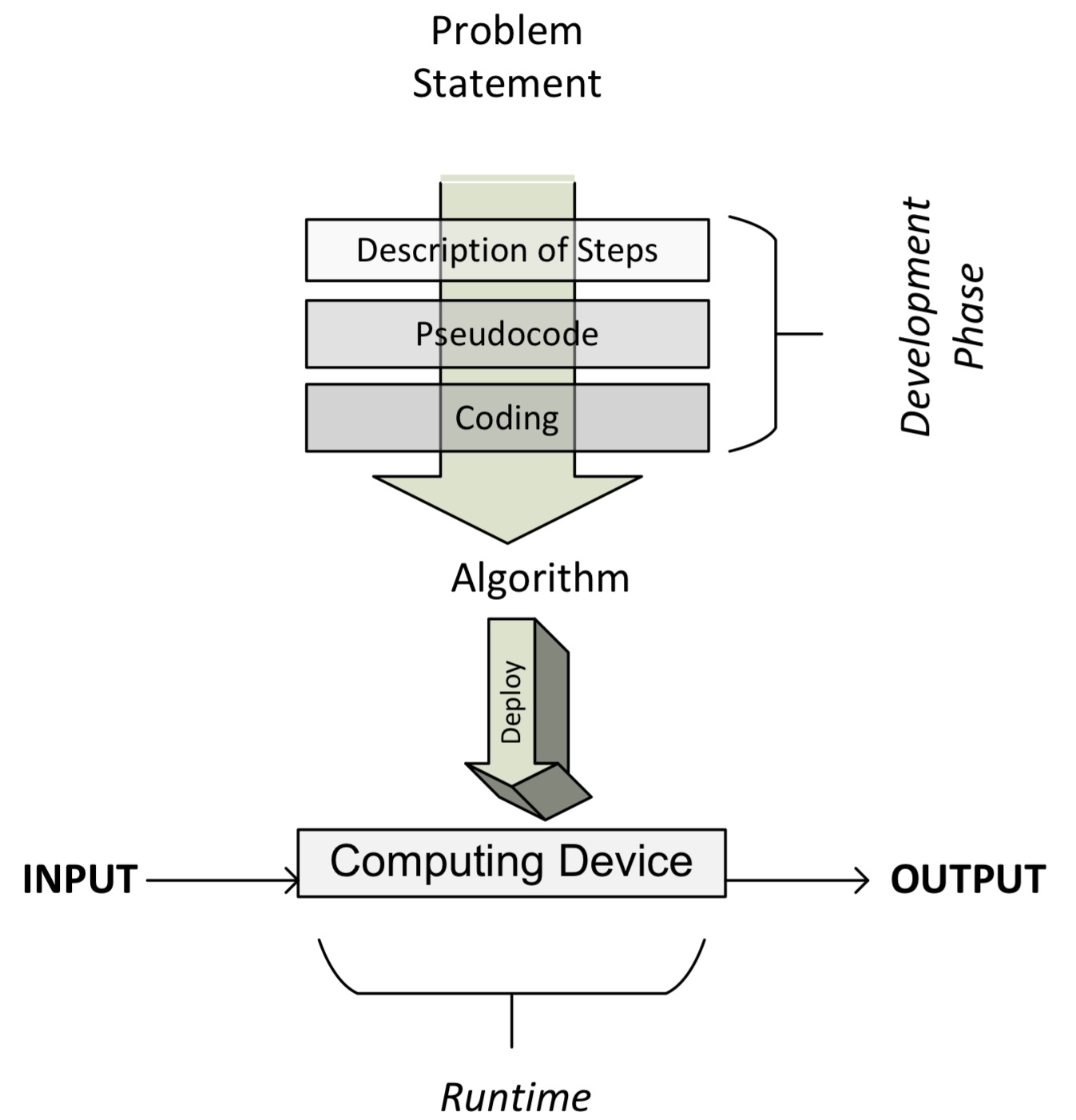Note that once the pseudocode is written (as we will see in the next section), we are ready to code the algorithm using the programming language of our choice.

# A practical example of pseudocode

Figure 1.3 shows the pseudocode of a resource allocation algorithm called SRPMP. In cluster computing, there are many situations where there are parallel tasks that need to be run on a set of available resources, collectively called a resource pool. This algorithm assigns tasks to a resource and creates a mapping set, called Ω. Note that the presented pseudocode captures the logic and flow of the algorithm, which is further explained in the following section:

`1: BEGIN Mapping_Phase2: Ω = { }3: k = 14: FOREACH Ti∈T5:     ωi = RA(Δk,Ti)6:     add {ωi,Ti} to Ω7:     state_changeTi [STATE 0: Idle/Unmapped] → [STATE 1: Idle/Mapped]8:     k=k+19:     IF (k>q)10:       k=111:    ENDIF12: END FOREACH13: END Mapping_Phase`

Let's parse this algorithm line by line:

1. We start the mapping by executing the algorithm. The Ω mapping set is empty.

2. The first partition is selected as the resource pool for the T1 task (see line 3 of the preceding code). Television Rating Point (TRPS) iteratively calls the Rheumatoid Arthritis (RA) algorithm for each Ti task with one of the partitions chosen as the resource pool.

3. The RA algorithm returns the set of resources chosen for the Ti task, represented by ωi (see line 5 of the preceding code).

4. Ti and ωi are added to the mapping set (see line 6 of the preceding code).

5. The state of Ti is changed from STATE 0:Idle/Mapping to STATE 1:Idle/Mapped (see line 7 of the preceding code).

6. Note that for the first iteration, k=1 and the first partition is selected. For each subsequent iteration, the value of k is increased until k>q.

7. If k becomes greater than q, it is reset to 1 again (see lines 9 and 10 of the preceding code).

8. This process is repeated until a mapping between all tasks and the set of resources they will use is determined and stored in a mapping set called Ω.

9. Once each of the tasks is mapped to a set of the resources in the mapping phase, it is executed.

# Using snippets

With the popularity of simple but powerful coding language such as Python, an alternative approach is becoming popular, which is to represent the logic of the algorithm directly in the programming language in a somewhat simplified version. Like pseudocode, this selected code captures the important logic and structure of the proposed algorithm, avoiding detailed code. This selected code is sometimes called a snippet. In this book, snippets are used instead of pseudocode wherever possible as they save one additional step. For example, let's look at a simple snippet that is about a Python function that can be used to swap two variables:

`define swap(x, y)    buffer = x    x = y    y = buffer`
Note that snippets cannot always replace pseudocode. In pseudocode, sometimes we abstract many lines of code as one line of pseudocode, expressing the logic of the algorithm without becoming distracted by unnecessary coding details.

# Creating an execution plan

Pseudocode and snippets are not always enough to specify all the logic related to more complex distributed algorithms. For example, distributed algorithms usually need to be divided into different coding phases at runtime that have a precedence order. The right strategy to divide the larger problem into an optimal number of phases with the right precedence constraints is crucial for the efficient execution of an algorithm.

We need to find a way to represent this strategy as well to completely represent the logic and structure of an algorithm. An execution plan is one of the ways of detailing how the algorithm will be subdivided into a bunch of tasks. A task can be mappers or reducers that can be grouped together in blocks called stages. The following diagram shows an execution plan that is generated by an Apache Spark runtime before executing an algorithm. It details the runtime tasks that the job created for executing our algorithm will be divided into: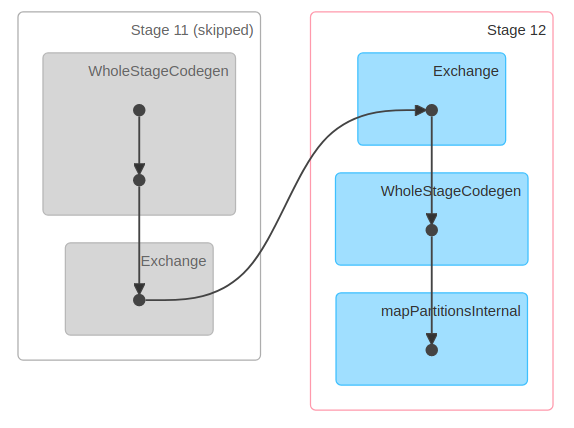Note that the preceding diagram has five tasks that have been divided into two different stages: Stage 11 and Stage 12.

# Introducing Python packages

Once designed, algorithms need to be implemented in a programming language as per the design. For this book, I chose the programming language Python. I chose it because Python is a flexible and open source programming language. Python is also the language of choice for increasingly important cloud computing infrastructures, such as Amazon Web Services (AWS), Microsoft Azure, and Google Cloud Platform (GCP).

The official Python home page is available at https://www.python.org/, which also has instructions for installation and a useful beginner's guide.

If you have not used Python before, it is a good idea to browse through this beginner's guide to self-study. A basic understanding of Python will help you to better understand the concepts presented in this book.

For this book, I expect you to use the recent version of Python 3. At the time of writing, the most recent version is 3.7.3, which is what we will use to run the exercises in this book.

# Python packages

Python is a general-purpose language. It is designed in a way that comes with bare minimum functionality. Based on the use case that you intend to use Python for, additional packages need to be installed. The easiest way to install additional packages is through the pip installer program. This pip command can be used to install the additional packages:

`pip install a_package`

The packages that have already been installed need to be periodically updated to get the latest functionality. This is achieved by using the upgrade flag:

`pip install a_package --upgrade`

Another Python distribution for scientific computing is Anaconda, which can be downloaded from http://continuum.io/downloads.

In addition to using the pip command to install new packages, for Anaconda distribution, we also have the option of using the following command to install new packages:

`conda install a_package`

To update the existing packages, the Anaconda distribution gives us the option to use the following command:

`conda update a_package`

There are all sorts of Python packages that are available. Some of the important packages that are relevant for algorithms are described in the following section.

# The SciPy ecosystem

Scientific Python (SciPy)—pronounced sigh pie—is a group of Python packages created for the scientific community. It contains many functions, including a wide range of random number generators, linear algebra routines, and optimizers. SciPy is a comprehensive package and, over time, people have developed many extensions to customize and extend the package according to their needs.

The following are the main packages that are part of this ecosystem:

• NumPy: For algorithms, the ability to create multi-dimensional data structures, such as arrays and matrices, is really important. NumPy offers a set of array and matrix data types that are important for statistics and data analysis. Details about NumPy can be found at http://www.numpy.org/.

• scikit-learn: This machine learning extension is one of the most popular extensions of SciPy. Scikit-learn provides a wide range of important machine learning algorithms, including classification, regression, clustering, and model validation. You can find more details about scikit-learn at http://scikit-learn.org/.

• pandas: pandas is an open source software library. It contains the tabular complex data structure that is used widely to input, output, and process tabular data in various algorithms. The pandas library contains many useful functions and it also offers highly optimized performance. More details about pandas can be found at http://pandas.pydata.org/.

• Matplotlib: Matplotlib provides tools to create powerful visualizations. Data can be presented as line plots, scatter plots, bacharts, histograms, pie charts, and so on. More information can be found at https://matplotlib.org/.

• Seaborn: Seaborn can be thought of as similar to the popular ggplot2 library in R. It is based on Matplotlib and offers an advanced interface for drawing brilliant statistical graphics. Further details can be found at https://seaborn.pydata.org/.

• iPython: iPython is an enhanced interactive console that is designed to facilitate the writing, testing, and debugging of Python code.

• Running Python programs: An interactive mode of programming is useful for learning and experimenting with code. Python programs can be saved in a text file with the .py extension and that file can be run from the console.

# Implementing Python via the Jupyter Notebook

Another way to run Python programs is through the Jupyter Notebook. The Jupyter Notebook provides a browser-based user interface to develop code. The Jupyter Notebook is used to present the code examples in this book. The ability to annotate and describe the code with texts and graphics makes it the perfect tool for presenting and explaining an algorithm and a great tool for learning.

To start the notebook, you need to start the Juypter-notebook process and then open your favorite browser and navigate to http://localhost:8888: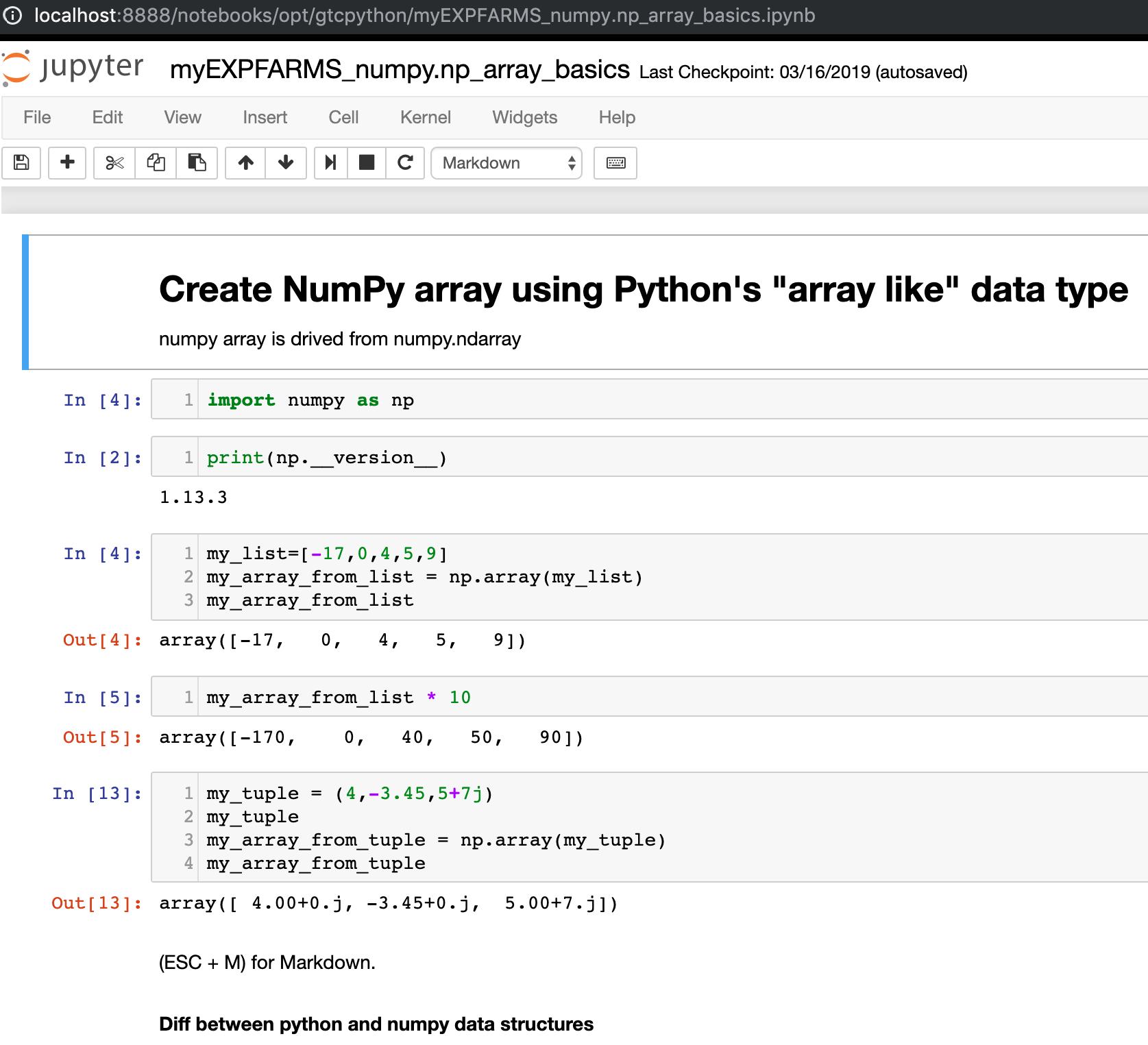Note that a Jupyter Notebook consists of different blocks called cells

# Algorithm design techniques

An algorithm is a mathematical solution to a real-world problem. When designing an algorithm, we keep the following three design concerns in mind as we work on designing and fine-tuning the algorithms:

• Concern 1: Is this algorithm producing the result we expected?

• Concern 2: Is this the most optimal way to get these results?

• Concern 3: How is the algorithm going to perform on larger datasets?

It is important to better understand the complexity of the problem itself before designing a solution for it. For example, it helps us to design an appropriate solution if we characterize the problem in terms of its needs and complexity. Generally, the algorithms can be divided into the following types based on the characteristics of the problem:

• Data-intensive algorithms: Data-intensive algorithms are designed to deal with a large amount of data. They are expected to have relatively simplistic processing requirements. A compression algorithm applied to a huge file is a good example of data-intensive algorithms. For such algorithms, the size of the data is expected to be much larger than the memory of the processing engine (a single node or cluster) and an iterative processing design may need to be developed to efficiently process the data according to the requirements.

• Compute-intensive algorithms: Compute-intensive algorithms have considerable processing requirements but do not involve large amounts of data. A simple example is the algorithm to find a very large prime number. Finding a strategy to divide the algorithm into different phases so that at least some of the phases are parallelized is key to maximizing the performance of the algorithm.

• Both data and compute-intensive algorithms: There are certain algorithms that deal with a large amount of data and also have considerable computing requirements. Algorithms used to perform sentiment analysis on live video feeds are a good example of where both the data and the processing requirements are huge in accomplishing the task. Such algorithms are the most resource-intensive algorithms and require careful design of the algorithm and intelligent allocation of available resources.

To characterize the problem in terms of its complexity and needs, it helps if we study its data and compute dimensions in more depth, which we will do in the following section.

# The data dimension

To categorize the data dimension of the problem, we look at its volume, velocity, and variety (the 3Vs), which are defined as follows:

• Volume: The volume is the expected size of the data that the algorithm will process.

• Velocity: The velocity is the expected rate of new data generation when the algorithm is used. It can be zero.

• Variety: The variety quantifies how many different types of data the designed algorithm is expected to deal with.

The following figure shows the 3Vs of the data in more detail. The center of this diagram shows the simplest possible data, with a small volume and low variety and velocity. As we move away from the center, the complexity of the data increases. It can increase in one or more of the three dimensions. For example, in the dimension of velocity, we have the Batch process as the simplest, followed by the Periodic process, and then the Near Real-Time process. Finally, we have the Real-Time process, which is the most complex to handle in the context of data velocity. For example, a collection of live video feeds gathered by a group of monitoring cameras will have a high volume, high velocity, and high variety and may need an appropriate design to have the ability to store and process data effectively. On the other hand, a simple .csv file created in Excel will have a low volume, low velocity, and low variety: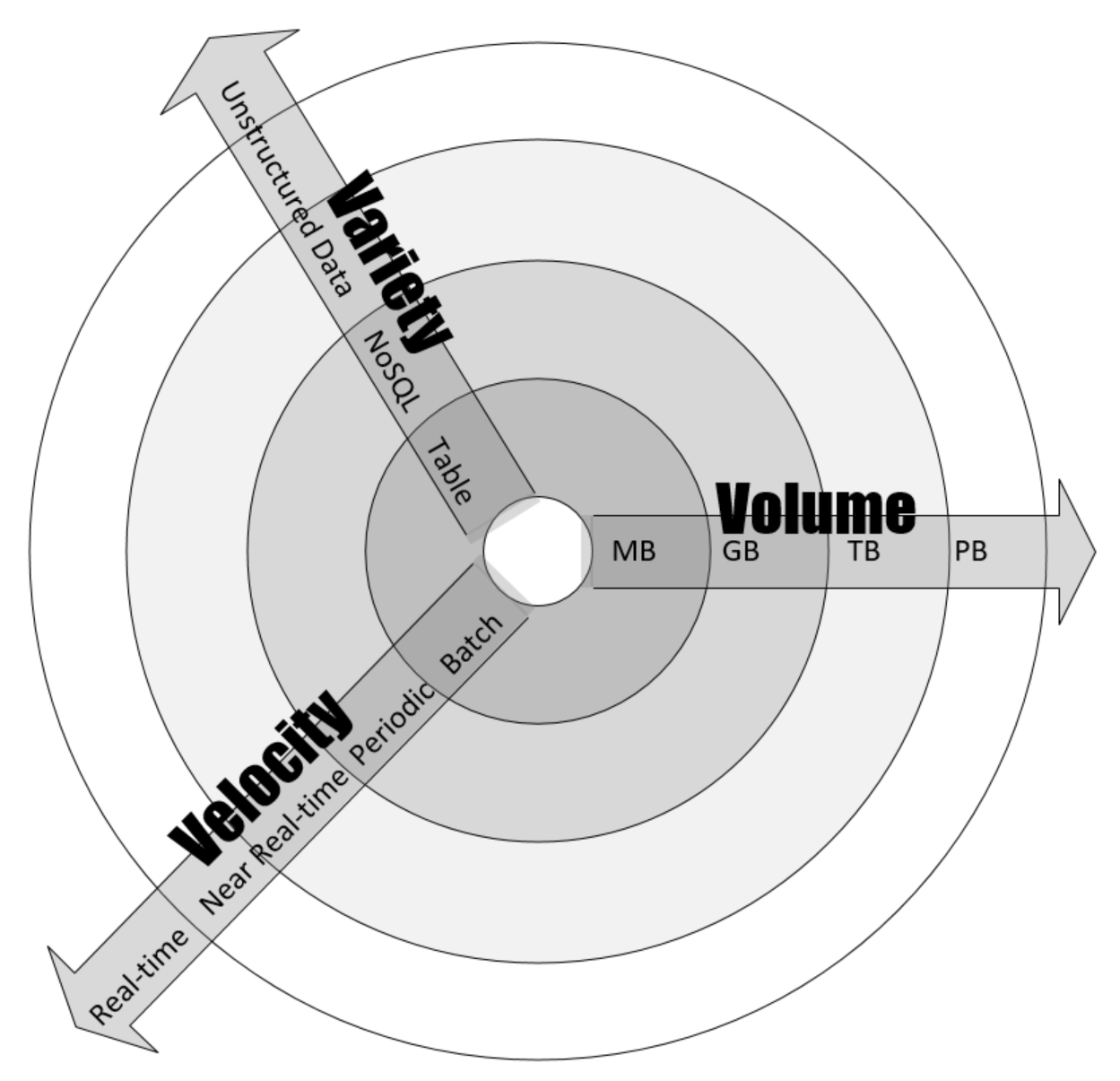For example, if the input data is a simple csv file, then the volume, velocity, and variety of the data will be low. On the other hand, if the input data is the live stream of a security video camera, then the volume, velocity, and variety of the data will be quite high and this problem should be kept in mind while designing an algorithm for it.

# Compute dimension

The compute dimension is about the processing and computing needs of the problem at hand. The processing requirements of an algorithm will determine what sort of design is most efficient for it. For example, deep learning algorithms, in general, require lots of processing power. It means that for deep learning algorithms, it is important to have multi-node parallel architecture wherever possible.

# A practical example

Let's assume that we want to conduct sentiment analysis on a video. Sentiment analysis is where we try to flag different portions of a video with human emotions of sadness, happiness, fear, joy, frustration, and ecstasy. It is a compute-intensive job where lots of computing power is needed. As you will see in the following figure, to design the compute dimension, we have divided the processing into five tasks, consisting of two stages. All the data transformation and preparation is implemented in three mappers. For that, we divide the video into three different partitions, called splits. After the mappers are executed, the resulting processed video is inputted to the two aggregators, called reducers. To conduct the required sentiment analysis, the reducers group the video according to the emotions. Finally, the results are combined in the output: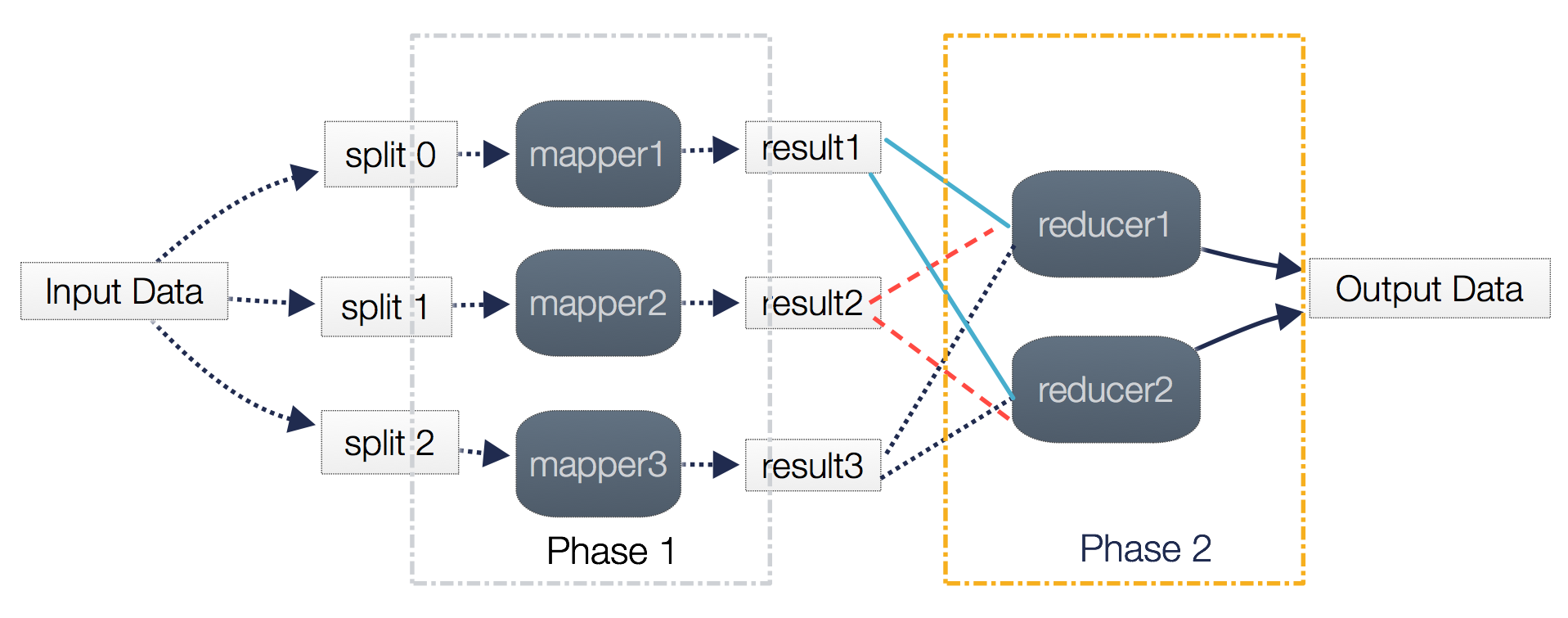Note that the number of mappers directly translates to the runtime parallelism of the algorithm. The optimal number of mappers and reducers is dependent on the characteristics of the data, the type of algorithm that is needed to be used, and the number of resources available.

# Performance analysis

Analyzing the performance of an algorithm is an important part of its design. One of the ways to estimate the performance of an algorithm is to analyze its complexity.

Complexity theory is the study of how complicated algorithms are. To be useful, any algorithm should have three key features:

• It should be correct. An algorithm won't do you much good if it doesn't give you the right answers.

• A good algorithm should be understandable. The best algorithm in the world won't do you any good if it's too complicated for you to implement on a computer.

• A good algorithm should be efficient. Even if an algorithm produces a correct result, it won't help you much if it takes a thousand years or if it requires 1 billion terabytes of memory.

There are two possible types of analysis to quantify the complexity of an algorithm:

• Space complexity analysis: Estimates the runtime memory requirements needed to execute the algorithm.

• Time complexity analysisEstimates the time the algorithm will take to run.

# Space complexity analysis

Space complexity analysis estimates the amount of memory required by the algorithm to process input data. While processing the input data, the algorithm needs to store the transient temporary data structures in memory. The way the algorithm is designed affects the number, type, and size of these data structures. In an age of distributed computing and with increasingly large amounts of data that needs to be processed, space complexity analysis is becoming more and more important. The size, type, and number of these data structures will dictate the memory requirements for the underlying hardware. Modern in-memory data structures used in distributed computing—such as Resilient Distributed Datasets (RDDs)—need to have efficient resource allocation mechanisms that are aware of the memory requirements at different execution phases of the algorithm.

Space complexity analysis is a must for the efficient design of algorithms. If proper space complexity analysis is not conducted while designing a particular algorithm, insufficient memory availability for the transient temporary data structures may trigger unnecessary disk spillovers, which could potentially considerably affect the performance and efficiency of the algorithm.

In this chapter, we will look deeper into time complexity. Space complexity will be discussed in Chapter 13, Large-Scale Algorithms, in more detail, where we will deal with large-scale distributed algorithms with complex runtime memory requirements.

# Time complexity analysis

Time complexity analysis estimates how long it will take for an algorithm to complete its assigned job based on its structure. In contrast to space complexity, time complexity is not dependent on any hardware that the algorithm will run on. Time complexity analysis solely depends on the structure of the algorithm itself. The overall goal of time complexity analysis is to try to answer these important questions—will this algorithm scale? How well will this algorithm handle larger datasets?

To answer these questions, we need to determine the effect on the performance of an algorithm as the size of the data is increased and make sure that the algorithm is designed in a way that not only makes it accurate but also scales well. The performance of an algorithm is becoming more and more important for larger datasets in today's world of "big data."

In many cases, we may have more than one approach available to design the algorithm. The goal of conducting time complexity analysis, in this case, will be as follows:

"Given a certain problem and more than one algorithm, which one is the most efficient to use in terms of time efficiency?"

There can be two basic approaches to calculating the time complexity of an algorithm:

• A post-implementation profiling approach: In this approach, different candidate algorithms are implemented and their performance is compared.

• A pre-implementation theoretical approach: In this approach, the performance of each algorithm is approximated mathematically before running an algorithm.

The advantage of the theoretical approach is that it only depends on the structure of the algorithm itself. It does not depend on the actual hardware that will be used to run the algorithm, the choice of the software stack chosen at runtime, or the programming language used to implement the algorithm.

# Estimating the performance

The performance of a typical algorithm will depend on the type of the data given to it as an input. For example, if the data is already sorted according to the context of the problem we are trying to solve, the algorithm may perform blazingly fast. If the sorted input is used to benchmark this particular algorithm, then it will give an unrealistically good performance number, which will not be a true reflection of its real performance in most scenarios. To handle this dependency of algorithms on the input data, we have different types of cases to consider when conducting a performance analysis.

# The best case

In the best case, the data given as input is organized in a way that the algorithm will give its best performance. Best-case analysis gives the upper bound of the performance.

# The worst case

The second way to estimate the performance of an algorithm is to try to find the maximum possible time it will take to get the job done under a given set of conditions. This worst-case analysis of an algorithm is quite useful as we are guaranteeing that regardless of the conditions, the performance of the algorithm will always be better than the numbers that come out of our analysis. Worst-case analysis is especially useful for estimating the performance when dealing with complex problems with larger datasets. Worst-case analysis gives the lower bound of the performance of the algorithm.

# The average case

This starts by dividing the various possible inputs into various groups. Then, it conducts the performance analysis from one of the representative inputs from each group. Finally, it calculates the average of the performance of each of the groups.

Average-case analysis is not always accurate as it needs to consider all the different combinations and possibilities of input to the algorithm, which is not always easy to do.

# Selecting an algorithm

How do you know which one is a better solution? How do you know which algorithm runs faster? Time complexity and Big O notation (discussed later in this chapter) are really good tools for answering these types of questions.

To see where it can be useful, let's take a simple example where the objective is to sort a list of numbers. There are a couple of algorithms available that can do the job. The issue is how to choose the right one.

First, an observation that can be made is that if there are not too many numbers in the list, then it does not matter which algorithm do we choose to sort the list of numbers. So, if there are only 10 numbers in the list (n=10), then it does not matter which algorithm we choose as it would probably not take more than a few microseconds, even with a very badly designed algorithm. But as soon as the size of the list becomes 1 million, now the choice of the right algorithm will make a difference. A very badly written algorithm might even take a couple of hours to run, while a well-designed algorithm may finish sorting the list in a couple of seconds. So, for larger input datasets, it makes a lot of sense to invest time and effort, perform a performance analysis, and choose the correctly designed algorithm that will do the job required in an efficient manner.

# Big O notation

Big O notation is used to quantify the performance of various algorithms as the input size grows. Big O notation is one of the most popular methodologies used to conduct worst-case analysis. The different kinds of Big O notation types are discussed in this section.

# Constant time (O(1)) complexity

If an algorithm takes the same amount of time to run, independent of the size of the input data, it is said to run in constant time. It is represented by O(1). Let's take the example of accessing the nth element of an array. Regardless of the size of the array, it will take constant time to get the results. For example, the following function will return the first element of the array and has a complexity of O(1):

`def getFirst(myList):    return myList`

The output is shown as: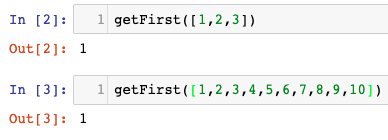• Addition of a new element to a stack by using push or removing an element from a stack by using pop. Regardless of the size of the stack, it will take the same time to add or remove an element.

• Accessing the element of the hashtable (as discussed in Chapter 2Data Structures Used in Algorithms).

• Bucket sort (as discussed in Chapter 2, Data Structures Used in Algorithms).

# Linear time (O(n)) complexity

An algorithm is said to have a complexity of linear time, represented by O(n), if the execution time is directly proportional to the size of the input. A simple example is to add the elements in a single-dimensional data structure:

`def getSum(myList):    sum = 0    for item in myList:        sum = sum + item    return sum`

Note the main loop of the algorithm. The number of iterations in the main loop increases linearly with an increasing value of n, producing an O(n) complexity in the following figure: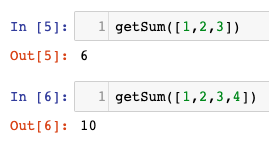Some other examples of array operations are as follows:

• Searching an element

• Finding the minimum value among all the elements of an array

# Quadratic time (O(n2)) complexity

An algorithm is said to run in quadratic time if the execution time of an algorithm is proportional to the square of the input size; for example, a simple function that sums up a two-dimensional array, as follows:

`def getSum(myList):    sum = 0    for row in myList:        for item in row:            sum += item    return sum`

Note the nested inner loop within the other main loop. This nested loop gives the preceding code the complexity of O(n2):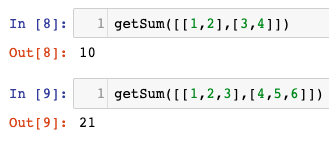Another example is the bubble sort algorithm (as discussed in Chapter 2Data Structures Used in Algorithms).

# Logarithmic time (O(logn)) complexity

An algorithm is said to run in logarithmic time if the execution time of the algorithm is proportional to the logarithm of the input size. With each iteration, the input size decreases by a constant multiple factor. An example of logarithmic is binary search. The binary search algorithm is used to find a particular element in a one-dimensional data structure, such as a Python list. The elements within the data structure need to be sorted in descending order. The binary search algorithm is implemented in a function named searchBinary, as follows:

`def searchBinary(myList,item):    first = 0    last = len(myList)-1    foundFlag = False    while( first<=last and not foundFlag):        mid = (first + last)//2        if myList[mid] == item :            foundFlag = True        else:            if item < myList[mid]:                last = mid - 1            else:                first = mid + 1    return foundFlag`

The main loop takes advantage of the fact that the list is ordered. It divides the list in half with each iteration until it gets to the result: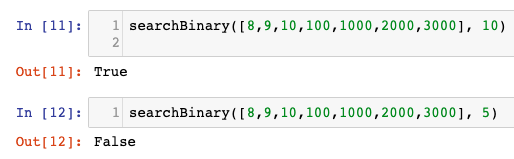After defining the function, it is tested to search a particular element in lines 11 and 12. The binary search algorithm is further discussed in Chapter 3, Sorting and Searching Algorithms.

Note that among the four types of Big O notation types presented, O(n2) has the worst performance and O(logn) has the best performance. In fact, O(logn)'s performance can be thought of as the gold standard for the performance of any algorithm (which is not always achieved, though). On the other hand, O(n2) is not as bad as O(n3) but still, algorithms that fall in this class cannot be used on big data as the time complexity puts limitations on how much data they can realistically process.

One way to reduce the complexity of an algorithm is to compromise on its accuracy, producing a type of algorithm called an approximate algorithm

The whole process of the performance evaluation of algorithms is iterative in nature, as shown in the following figure: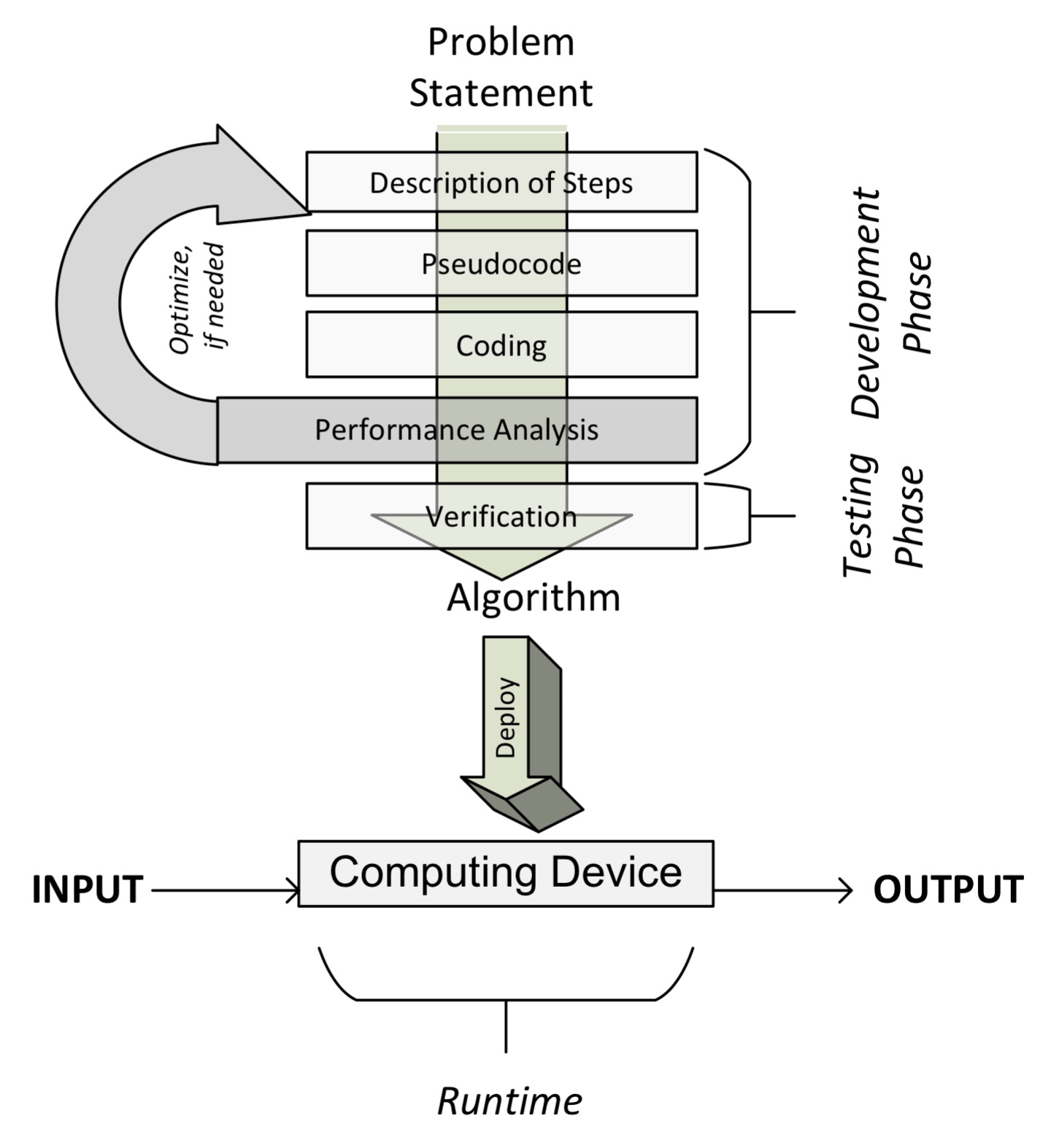# Validating an algorithm

Validating an algorithm confirms that it is actually providing a mathematical solution to the problem we are trying to solve. A validation process should check the results for as many possible values and types of input values as possible.

# Exact, approximate, and randomized algorithms

Validating an algorithm also depends on the type of the algorithm as the testing techniques are different. Let's first differentiate between deterministic and randomized algorithms.

For deterministic algorithms, a particular input always generates exactly the same output. But for certain classes of algorithms, a sequence of random numbers is also taken as input, which makes the output different each time the algorithm is run. The k-means clustering algorithm, which is detailed in Chapter 6Unsupervised Machine Learning Algorithms, is an example of such an algorithm: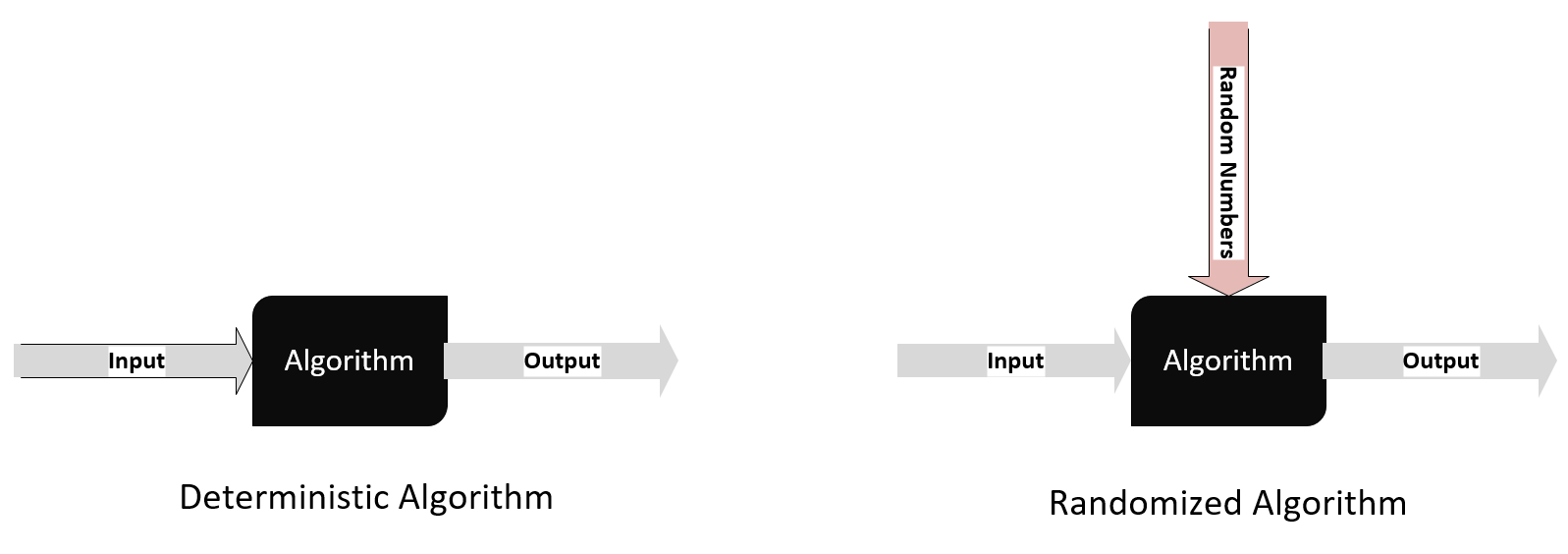Algorithms can also be divided into the following two types based on assumptions or approximation used to simplify the logic to make them run faster:

• An exact algorithm: Exact algorithms are expected to produce a precise solution without introducing any assumptions or approximations.

•  An approximate algorithm: When the problem complexity is too much to handle for the given resources, we simplify our problem by making some assumptions. The algorithms based on these simplifications or assumptions are called approximate algorithms, which doesn't quite give us the precise solution.

Let's look at an example to understand the difference between the exact and approximate algorithms—the famous traveling salesman problem, which was presented in 1930. A traveling salesman challenges you to find the shortest route for a particular salesman that visits each city (from a list of cities) and then returns to the origin, which is why he is named the traveling salesman. The first attempt to provide the solution will include generating all the permutations of cities and choosing the combination of cities that is cheapest. The complexity of this approach to provide the solution is O(n!), where n is the number of cities. It is obvious that time complexity starts to become unmanageable beyond 30 cities.

If the number of cities is more than 30, one way of reducing the complexity is to introduce some approximations and assumptions.

For approximate algorithms, it is important to set the expectations for accuracy when gathering the requirements. Validating an approximation algorithm is about verifying that the error of the results is within an acceptable range.

# Explainability

When algorithms are used for critical cases, it becomes important to have the ability to explain the reason behind each and every result whenever needed. This is necessary to make sure that decisions based on the results of the algorithms do not introduce bias.

The ability to exactly identify the features that are used directly or indirectly to come up with a particular decision is called the explainability of an algorithm. Algorithms, when used for critical use cases, need to be evaluated for bias and prejudice. The ethical analysis of algorithms has become a standard part of the validation process for those algorithms that can affect decision-making that relates to the life of people.

For algorithms that deal with deep learning, explainability is difficult to achieve. For example, if an algorithm is used to refuse the mortgage application of a person, it is important to have the transparency and ability to explain the reason.

Algorithmic explainability is an active area of research. One of the effective techniques that has been recently developed is Local Interpretable Model-Agnostic Explanations (LIME), as proposed in the proceedings of the 22nd Association for Computing Machinery (ACM) at thSpecial Interest Group on Knowledge Discovery (SIGKDD) international conference on knowledge discovery and data mining in 2016. LIME is based on a concept where small changes are induced to the input for each instance and then an effort to map the local decision boundary for that instance is made. It can then quantify the influence of each variable for that instance.

# Summary

This chapter was about learning the basics of algorithms. First, we learned about the different phases of developing an algorithm. We discussed the different ways of specifying the logic of an algorithm that are necessary for designing it. Then, we looked at how to design an algorithm. We learned two different ways of analyzing the performance of an algorithm. Finally, we studied different aspects of validating an algorithm.

After going through this chapter, we should be able to understand the pseudocode of an algorithm. We should understand the different phases in developing and deploying an algorithm. We also learned how to use Big O notation to evaluate the performance of an algorithm.

The next chapter is about the data structures used in algorithms. We will start by looking at the data structures available in Python. We will then look at how we can use these data structures to create more sophisticated data structures, such as stacks, queues, and trees, which are needed to develop complex algorithms.

### About the Author

• ##### Imran Ahmad

Imran Ahmad is a certified Google Instructor who has been teaching for Google and Learning Tree for the last many years. Imran completed his PhD in 2009 in which he proposed an AI used to develop intelligent clouds for Sudbury Neutrino Observatory. Since 2005 Imran is working in a high-profile machine learning project at the Advanced Analytics Lab of the Canadian Federal Government. The project is to develop machine learning algorithms that can automate the process of immigration. Currently Imran is also doing research on semi-supervised learning and edge computing

Browse publications by this author

### Latest Reviews

(20 reviews total)